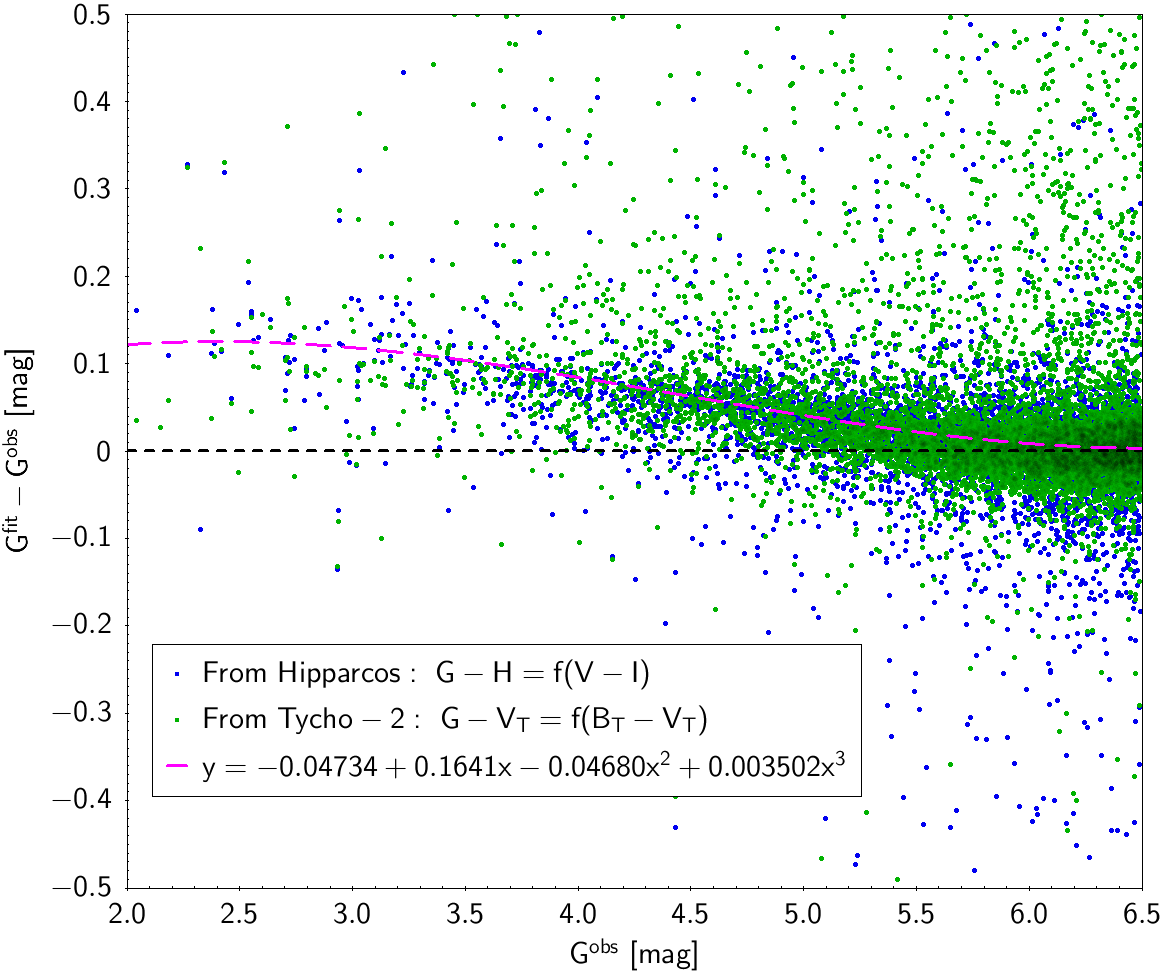# 5.3.8 Saturation correction

Author(s): Josep M. Carrasco

The current calibration is not perfectly accounting for saturation effects (see Figure 5.22 comparing residuals in the Hipparcos and Tycho-2 photometric transformations derived in Section 5.3.7 as a function of the magnitude).

In order to provide a tool to empirically correct this trend at bright magnitudes we fit here a relationship of this trend in Figure 5.22, combining both Hipparcos and Tycho-2 residuals. The resulting fitted relationships are the following:

 $\displaystyle G^{\rm corr}-G$ $\displaystyle=$ $\displaystyle-0.047344+0.16405G-0.046799G^{2}+0.0035015G^{3}$ $\displaystyle G_{\rm BP}^{\rm corr}-G_{\rm BP}$ $\displaystyle=$ $\displaystyle-2.0384+0.95282G-0.11018G^{2}$ $\displaystyle G_{\rm RP}^{\rm corr}-G_{\rm RP}$ $\displaystyle=$ $\displaystyle-13.946+13.239G_{\rm RP}-4.23G_{\rm RP}^{2}+0.4532G_{\rm RP}^{3}$

The ranges of applicability for these relationships are the following:

• $2.0 mag for the $G$ relationship,

• $2.0 mag for the $G_{\rm BP}$ relationship, and

• $2.0 mag for the $G_{\rm RP}$ relationship.Figure 5.22: Residuals of the fitted laws for Hipparcos and Tycho-2 in Table 5.7 as a function of the magnitude for G (top), GBP (center) and GRP (bottom) (Evans et al. 2018)